Function Repository Resource:

# CompareDice

Given two dice, calculate the odds that the first will win, tie or lose

Contributed by: Ed Pegg Jr
 ResourceFunction["CompareDice"][a, b] calculate the odds that die a will {win, tie, lose} to die b.

## Examples

### Basic Examples (1)

A 4-sided die has a 1/4 chance of rolling higher than a 6-sided die:

 In:=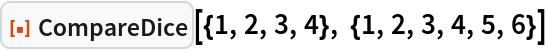Out=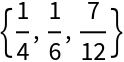### Scope (4)

In these intransitive dice, a>b>c>a:

 In:=Out=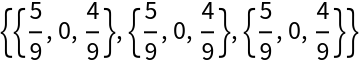In Efron's dice, a>b>c>d>a:

 In:=Out=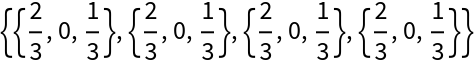With Miwin's dice, a>b>c>a:

 In:=Out=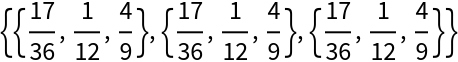A new set of intransitive dice:

 In:=Out=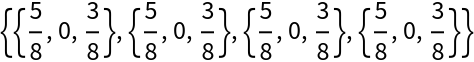### Neat Examples (2)

Any two of Eric Harshbarger's "Go First Dice" have equal odds of winning:

 In:=Out=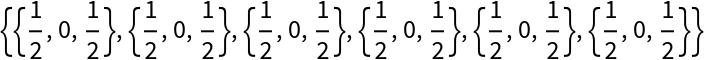In this set of fooling dice, a>b>c>d>e>a:

 In:=Out=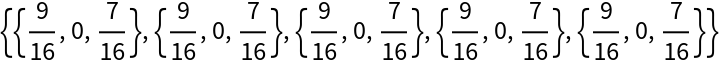However, if pairs of the dice are rolled, a<b<c<d<e<a:

 In:=Out=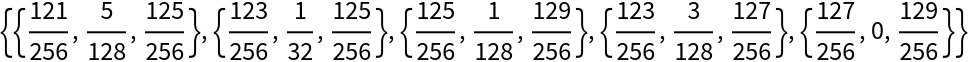## Version History

• 1.0.0 – 08 December 2022